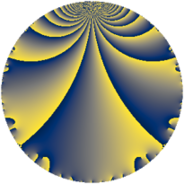# Properties

 Label 3549.2.a.cLevel $3549$ Weight $2$ Character orbit 3549.a Self dual yes Analytic conductor $28.339$ Analytic rank $1$ Dimension $1$ CM no Inner twists $1$

# Related objects

## Newspace parameters

 Level: $$N$$ $$=$$ $$3549 = 3 \cdot 7 \cdot 13^{2}$$ Weight: $$k$$ $$=$$ $$2$$ Character orbit: $$[\chi]$$ $$=$$ 3549.a (trivial)

## Newform invariants

 Self dual: yes Analytic conductor: $$28.3389076774$$ Analytic rank: $$1$$ Dimension: $$1$$ Coefficient field: $$\mathbb{Q}$$ Coefficient ring: $$\mathbb{Z}$$ Coefficient ring index: $$1$$ Twist minimal: no (minimal twist has level 21) Fricke sign: $$1$$ Sato-Tate group: $\mathrm{SU}(2)$

## $q$-expansion

 $$f(q)$$ $$=$$ $$q + q^{2} + q^{3} - q^{4} + 2q^{5} + q^{6} + q^{7} - 3q^{8} + q^{9} + O(q^{10})$$ $$q + q^{2} + q^{3} - q^{4} + 2q^{5} + q^{6} + q^{7} - 3q^{8} + q^{9} + 2q^{10} - 4q^{11} - q^{12} + q^{14} + 2q^{15} - q^{16} - 6q^{17} + q^{18} - 4q^{19} - 2q^{20} + q^{21} - 4q^{22} - 3q^{24} - q^{25} + q^{27} - q^{28} - 2q^{29} + 2q^{30} + 5q^{32} - 4q^{33} - 6q^{34} + 2q^{35} - q^{36} - 6q^{37} - 4q^{38} - 6q^{40} - 2q^{41} + q^{42} - 4q^{43} + 4q^{44} + 2q^{45} - q^{48} + q^{49} - q^{50} - 6q^{51} + 6q^{53} + q^{54} - 8q^{55} - 3q^{56} - 4q^{57} - 2q^{58} - 12q^{59} - 2q^{60} - 2q^{61} + q^{63} + 7q^{64} - 4q^{66} - 4q^{67} + 6q^{68} + 2q^{70} - 3q^{72} + 6q^{73} - 6q^{74} - q^{75} + 4q^{76} - 4q^{77} - 16q^{79} - 2q^{80} + q^{81} - 2q^{82} + 12q^{83} - q^{84} - 12q^{85} - 4q^{86} - 2q^{87} + 12q^{88} + 14q^{89} + 2q^{90} - 8q^{95} + 5q^{96} - 18q^{97} + q^{98} - 4q^{99} + O(q^{100})$$

## Embeddings

For each embedding $$\iota_m$$ of the coefficient field, the values $$\iota_m(a_n)$$ are shown below.

For more information on an embedded modular form you can click on its label.

Label $$\iota_m(\nu)$$ $$a_{2}$$ $$a_{3}$$ $$a_{4}$$ $$a_{5}$$ $$a_{6}$$ $$a_{7}$$ $$a_{8}$$ $$a_{9}$$ $$a_{10}$$
1.1
 0
1.00000 1.00000 −1.00000 2.00000 1.00000 1.00000 −3.00000 1.00000 2.00000
 $$n$$: e.g. 2-40 or 990-1000 Significant digits: Format: Complex embeddings Normalized embeddings Satake parameters Satake angles

## Atkin-Lehner signs

$$p$$ Sign
$$3$$ $$-1$$
$$7$$ $$-1$$
$$13$$ $$1$$

## Inner twists

This newform does not admit any (nontrivial) inner twists.

## Twists

By twisting character orbit
Char Parity Ord Mult Type Twist Min Dim
1.a even 1 1 trivial 3549.2.a.c 1
13.b even 2 1 21.2.a.a 1
39.d odd 2 1 63.2.a.a 1
52.b odd 2 1 336.2.a.a 1
65.d even 2 1 525.2.a.d 1
65.h odd 4 2 525.2.d.a 2
91.b odd 2 1 147.2.a.a 1
91.r even 6 2 147.2.e.b 2
91.s odd 6 2 147.2.e.c 2
104.e even 2 1 1344.2.a.g 1
104.h odd 2 1 1344.2.a.s 1
117.n odd 6 2 567.2.f.b 2
117.t even 6 2 567.2.f.g 2
143.d odd 2 1 2541.2.a.j 1
156.h even 2 1 1008.2.a.l 1
195.e odd 2 1 1575.2.a.c 1
195.s even 4 2 1575.2.d.a 2
208.o odd 4 2 5376.2.c.l 2
208.p even 4 2 5376.2.c.r 2
221.b even 2 1 6069.2.a.b 1
247.d odd 2 1 7581.2.a.d 1
260.g odd 2 1 8400.2.a.bn 1
273.g even 2 1 441.2.a.f 1
273.w odd 6 2 441.2.e.a 2
273.ba even 6 2 441.2.e.b 2
312.b odd 2 1 4032.2.a.h 1
312.h even 2 1 4032.2.a.k 1
364.h even 2 1 2352.2.a.v 1
364.x even 6 2 2352.2.q.e 2
364.bl odd 6 2 2352.2.q.x 2
429.e even 2 1 7623.2.a.g 1
455.h odd 2 1 3675.2.a.n 1
728.b even 2 1 9408.2.a.m 1
728.l odd 2 1 9408.2.a.bv 1
1092.d odd 2 1 7056.2.a.p 1

By twisted newform orbit
Twist Min Dim Char Parity Ord Mult Type
21.2.a.a 1 13.b even 2 1
63.2.a.a 1 39.d odd 2 1
147.2.a.a 1 91.b odd 2 1
147.2.e.b 2 91.r even 6 2
147.2.e.c 2 91.s odd 6 2
336.2.a.a 1 52.b odd 2 1
441.2.a.f 1 273.g even 2 1
441.2.e.a 2 273.w odd 6 2
441.2.e.b 2 273.ba even 6 2
525.2.a.d 1 65.d even 2 1
525.2.d.a 2 65.h odd 4 2
567.2.f.b 2 117.n odd 6 2
567.2.f.g 2 117.t even 6 2
1008.2.a.l 1 156.h even 2 1
1344.2.a.g 1 104.e even 2 1
1344.2.a.s 1 104.h odd 2 1
1575.2.a.c 1 195.e odd 2 1
1575.2.d.a 2 195.s even 4 2
2352.2.a.v 1 364.h even 2 1
2352.2.q.e 2 364.x even 6 2
2352.2.q.x 2 364.bl odd 6 2
2541.2.a.j 1 143.d odd 2 1
3549.2.a.c 1 1.a even 1 1 trivial
3675.2.a.n 1 455.h odd 2 1
4032.2.a.h 1 312.b odd 2 1
4032.2.a.k 1 312.h even 2 1
5376.2.c.l 2 208.o odd 4 2
5376.2.c.r 2 208.p even 4 2
6069.2.a.b 1 221.b even 2 1
7056.2.a.p 1 1092.d odd 2 1
7581.2.a.d 1 247.d odd 2 1
7623.2.a.g 1 429.e even 2 1
8400.2.a.bn 1 260.g odd 2 1
9408.2.a.m 1 728.b even 2 1
9408.2.a.bv 1 728.l odd 2 1

## Hecke kernels

This newform subspace can be constructed as the intersection of the kernels of the following linear operators acting on $$S_{2}^{\mathrm{new}}(\Gamma_0(3549))$$:

 $$T_{2} - 1$$ $$T_{5} - 2$$

## Hecke characteristic polynomials

$p$ $F_p(T)$
$2$ $$-1 + T$$
$3$ $$-1 + T$$
$5$ $$-2 + T$$
$7$ $$-1 + T$$
$11$ $$4 + T$$
$13$ $$T$$
$17$ $$6 + T$$
$19$ $$4 + T$$
$23$ $$T$$
$29$ $$2 + T$$
$31$ $$T$$
$37$ $$6 + T$$
$41$ $$2 + T$$
$43$ $$4 + T$$
$47$ $$T$$
$53$ $$-6 + T$$
$59$ $$12 + T$$
$61$ $$2 + T$$
$67$ $$4 + T$$
$71$ $$T$$
$73$ $$-6 + T$$
$79$ $$16 + T$$
$83$ $$-12 + T$$
$89$ $$-14 + T$$
$97$ $$18 + T$$